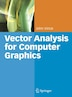# Vector Analysis for Computer Graphics

## byJohn Vince

October 13, 2010|\$150.50
Paperback
Earn 753 plum® points
Ships free on orders over \$35

In stock online

Not available in stores

Prices and offers may vary in store

In my last book, Geometry for Computer Graphics, I employed a mixture of algebra and vector analysis to prove many of the equations used in computer graphics. At the time, I did not make any distinction between the two methodologies, but slowly it dawned upon me that I had had to discover, for the first time, how to use vector analysis and associated strategies for solving geometric problems. I suppose that mathematicians are taught this as part of their formal mathematical training, but then, I am not a mathematician! After some deliberation, I decided to write a book that would introduce the beginner to the world of vectors and their application to the geometric problems encountered in computer graphics. I accepted the fact that there would be some duplication of formulas between this and my last book; however, this time I would concentrate on explaining how problems are solved. The book contains eleven chapters: The first chapter distinguishes between scalar and vector quantities, which is reasonably straightforward. The second chapter introduces vector repres- tation, starting with Cartesian coordinates and concluding with the role of direction cosines in changes in axial systems. The third chapter explores how the line equation has a natural vector interpretation and how vector analysis is used to resolve a variety of line-related, geometric problems. Chapter 4 repeats Chapter 3 in the context of the plane.
Scalars and Vectors.- Vector Representation.- Straight Lines.- The Plane.- Reflections.- Intersections.- Rotating Vectors.- Vector Differentiation.- Projections.- Rendering.- Motion.